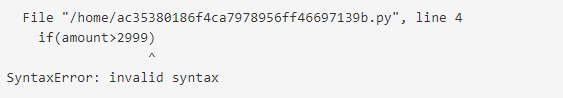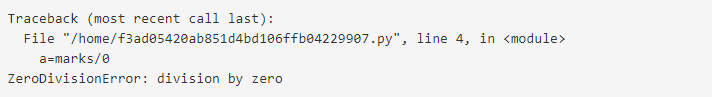# Python Exception Handling

• Difficulty Level : Easy
• Last Updated : 15 Jul, 2022

We have explored basic python till now from Set 1 to 4 (Set 1 | Set 2 | Set 3 | Set 4).

In this article, we will discuss how to handle exceptions in Python using try. catch, and finally statement with the help of proper examples.

Error in Python can be of two types i.e. Syntax errors and Exceptions. Errors are the problems in a program due to which the program will stop the execution. On the other hand, exceptions are raised when some internal events occur which changes the normal flow of the program.

## Difference between Syntax Error and Exceptions

Syntax Error: As the name suggests this error is caused by the wrong syntax in the code. It leads to the termination of the program.

Example:

## Python3

 `# initialize the amount variable ` `amount ``=` `10000` ` `  `# check that You are eligible to ` `#  purchase Dsa Self Paced or not ` `if``(amount > ``2999``) ` `print``(``"You are eligible to purchase Dsa Self Paced"``) `

Output:Exceptions: Exceptions are raised when the program is syntactically correct, but the code resulted in an error. This error does not stop the execution of the program, however, it changes the normal flow of the program.

Example:

## Python3

 `# initialize the amount variable ` `marks ``=` `10000` ` `  `# perform division with 0 ` `a ``=` `marks ``/` `0` `print``(a) `

Output:In the above example raised the ZeroDivisionError as we are trying to divide a number by 0.

Note: Exception is the base class for all the exceptions in Python. You can check the exception hierarchy here.

## Try and Except Statement – Catching Exceptions

Try and except statements are used to catch and handle exceptions in Python. Statements that can raise exceptions are kept inside the try clause and the statements that handle the exception are written inside except clause.

Example: Let us try to access the array element whose index is out of bound and handle the corresponding exception.

## Python3

 `# Python program to handle simple runtime error ` `#Python 3 ` ` `  `a ``=` `[``1``, ``2``, ``3``] ` `try``:  ` `    ``print` `(``"Second element = %d"` `%``(a[``1``])) ` ` `  `    ``# Throws error since there are only 3 elements in array ` `    ``print` `(``"Fourth element = %d"` `%``(a[``3``])) ` ` `  `except``: ` `    ``print` `(``"An error occurred"``) `

Output

```Second element = 2
An error occurred```

In the above example, the statements that can cause the error are placed inside the try statement (second print statement in our case). The second print statement tries to access the fourth element of the list which is not there and this throws an exception. This exception is then caught by the except statement.

## Catching Specific Exception

A try statement can have more than one except clause, to specify handlers for different exceptions. Please note that at most one handler will be executed. For example, we can add IndexError in the above code. The general syntax for adding specific exceptions are –

```try:
# statement(s)
except IndexError:
# statement(s)
except ValueError:
# statement(s)```

Example: Catching specific exception in Python

## Python3

 `# Program to handle multiple errors with one ` `# except statement ` `# Python 3 ` ` `  `def` `fun(a): ` `    ``if` `a < ``4``: ` ` `  `        ``# throws ZeroDivisionError for a = 3 ` `        ``b ``=` `a``/``(a``-``3``) ` ` `  `    ``# throws NameError if a >= 4 ` `    ``print``(``"Value of b = "``, b) ` `     `  `try``: ` `    ``fun(``3``) ` `    ``fun(``5``) ` ` `  `# note that braces () are necessary here for  ` `# multiple exceptions ` `except` `ZeroDivisionError: ` `    ``print``(``"ZeroDivisionError Occurred and Handled"``) ` `except` `NameError: ` `    ``print``(``"NameError Occurred and Handled"``)`

Output

`ZeroDivisionError Occurred and Handled`

If you comment on the line fun(3), the output will be

`NameError Occurred and Handled`

The output above is so because as soon as python tries to access the value of b, NameError occurs.

## Try with Else Clause

In python, you can also use the else clause on the try-except block which must be present after all the except clauses. The code enters the else block only if the try clause does not raise an exception.

Example: Try with else clause

## Python3

 `# Program to depict else clause with try-except ` `# Python 3 ` `# Function which returns a/b ` `def` `AbyB(a , b): ` `    ``try``: ` `        ``c ``=` `((a``+``b) ``/` `(a``-``b)) ` `    ``except` `ZeroDivisionError: ` `        ``print` `(``"a/b result in 0"``) ` `    ``else``: ` `        ``print` `(c) ` ` `  `# Driver program to test above function ` `AbyB(``2.0``, ``3.0``) ` `AbyB(``3.0``, ``3.0``) `

Output:

```-5.0
a/b result in 0 ```

## Finally Keyword in Python

Python provides a keyword finally, which is always executed after the try and except blocks. The final block always executes after normal termination of try block or after try block terminates due to some exception.

Syntax:

```try:
# Some Code....

except:
# optional block
# Handling of exception (if required)

else:
# execute if no exception

finally:
# Some code .....(always executed)```

Example:

## Python3

 `# Python program to demonstrate finally ` ` `  `# No exception Exception raised in try block ` `try``: ` `    ``k ``=` `5``/``/``0`  `# raises divide by zero exception. ` `    ``print``(k) ` ` `  `# handles zerodivision exception ` `except` `ZeroDivisionError: ` `    ``print``(``"Can't divide by zero"``) ` ` `  `finally``: ` `    ``# this block is always executed ` `    ``# regardless of exception generation. ` `    ``print``(``'This is always executed'``) `

Output:

```Can't divide by zero
This is always executed```

## Raising Exception

The raise statement allows the programmer to force a specific exception to occur. The sole argument in raise indicates the exception to be raised. This must be either an exception instance or an exception class (a class that derives from Exception).

## Python3

 `# Program to depict Raising Exception ` ` `  `try``:  ` `    ``raise` `NameError(``"Hi there"``)  ``# Raise Error ` `except` `NameError: ` `    ``print` `(``"An exception"``) ` `    ``raise`  `# To determine whether the exception was raised or not `

The output of the above code will simply line printed as “An exception” but a Runtime error will also occur in the last due to the raise statement in the last line. So, the output on your command line will look like

```Traceback (most recent call last):
File "/home/d6ec14ca595b97bff8d8034bbf212a9f.py", line 5, in <module>
raise NameError("Hi there")  # Raise Error
NameError: Hi there```